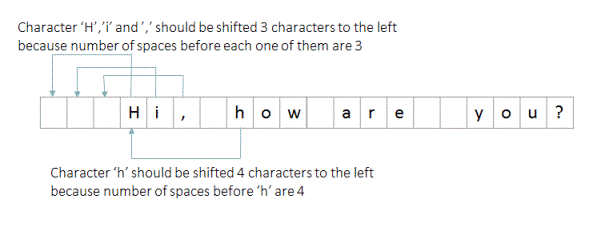# Remove spaces from a given string

Given a string of characters consisting of 0 or more spaces, remove all the spaces from this given string. Separation of spaces from other characters should be done in-place(without using extra space). Expected time complexity is O(n).

For example, if the input string is "  Hi, How are you?  " then the output returned should be "Hi,Howareyou?"

## Algorithm/Insights

For a given string, if there are 'j' number of spaces to the left of the character at index 'i', then that 'i'th character would be placed at (i-j)th index after removing all spaces from the given string.

Using above idea, all we do is traverse the given string from the leftmost character and keep counting the number of spaces seen so far. If the current character at index 'i' is a non-space character and if there are 'j' number of spaces seen so far then we copy 'i'th character to index (i-j). Note that because we are traversing this string from the leftmost end, character at index (i-j) would already have been placed in its correct position and therefore we don't need to worry about overwriting it. Following example should help you to visualize this step.After the traversal of complete string, we would have counted the total number of spaces in the given string, let that be 'numSpaces'. Now all we do is remove the last 'numSpaces' characters of this string by copying characters of this modified string from index 0 to index (lengthOfString - numSpaces - 1) including both extremes into a new string. You can refer to function 'removeSpaces(String str)' for implementation details.

Please note that in the Java programming language, unlike C, an array of char is not a String, and neither a String nor an array of char is terminated by '\u0000' (the NUL character). Reference:  https://docs.oracle.com/javase/specs/jls/se7/html/jls-10.html#jls-10.9 .  Therefore we have to copy the modified string into a new string which would take O(n) extra space.

## Code Snippet

```
package com.ideserve.nilesh.questions;

import java.util.Arrays;

/**
* <b>IDeserve <br>
* Removes spaces from a given string in O(n) time.
* @author Nilesh
*/

public class RemoveSpaces
{
// removes spaces from given string
public static String removeSpaces(String str)
{
char[] charArray = str.toCharArray();

int numSpaces = 0; // number of spaces before 'i'th character
for (int i = 0; i < charArray.length; i++)
{
// count number of spaces
if (charArray[i] == ' ')
{
numSpaces += 1;
}
// put 'i'th character into its correct position after removing spaces before it
else
{
charArray[i-numSpaces] = charArray[i];
}
}

// all the spaces are moved towards the end of the string.
// Create new string by using non-space characters
charArray = Arrays.copyOf(charArray, charArray.length - numSpaces);

return new String(charArray);
}

public static void main(String[] args)
{
String strWithSpaces    = "  Hi there, how  are you   doing? ";
String strWithoutSpaces = removeSpaces(strWithSpaces);

System.out.println(strWithoutSpaces);
}
}
```

## Order of the Algorithm

Time Complexity is O(n)
Space Complexity is O(n)